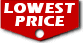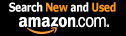# A Student's Guide to Maxwell's Equations (Student's Guides) / Fleisch, Daniel

List Price: \$31.99
Our Price: \$21.26For Bulk orders
Quantity

Used Book Price:
/ Fleisch, Daniel
Publisher: Cambridge University Press
Availability:In Stock.
Sales Rank: 50939
 ISBN-10: 0521701473ISBN-13: 9780521701471

Similar Books
 A Student's Guide to Waves (Student's Guides) A Student's Guide to Vectors and Tensors (Student's Guides) Div, Grad, Curl, and All That: An Informal Text on Vector Calculus (Fourth Edition) A Student's Guide to Lagrangians and Hamiltonians (Student's Guides) A Student's Guide to the Mathematics of Astronomy (Student's Guides) Schaum's Outline of Electromagnetics, 4th Edition (Schaum's Outlines) Introduction to Electrodynamics Vector Analysis, 2nd Edition Special Relativity and Classical Field Theory: The Theoretical Minimum A Student's Guide to Fourier Transforms: With Applications in Physics and Engineering (Student's Guides)

Gauss's law for electric fields, Gauss's law for magnetic fields, Faraday's law, and the Ampere-Maxwell law are four of the most influential equations in science. In this guide for students, each equation is the subject of an entire chapter, with detailed, plain-language explanations of the physical meaning of each symbol in the equation, for both the integral and differential forms. The final chapter shows how Maxwell's equations may be combined to produce the wave equation, the basis for the electromagnetic theory of light. This book is a wonderful resource for undergraduate and graduate courses in electromagnetism and electromagnetics. A website hosted by the author at www.cambridge.org/9780521701471 contains interactive solutions to every problem in the text as well as audio podcasts to walk students through each chapter.
Now you can buy Books online in USA,UK, India and more than 100 countries.
*Terms and Conditions apply
Disclaimer: All product data on this page belongs to.
No guarantees are made as to accuracy of prices and information.